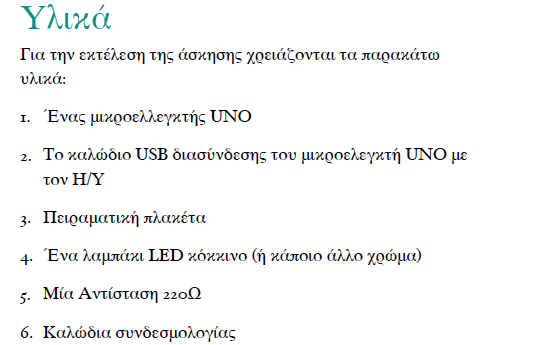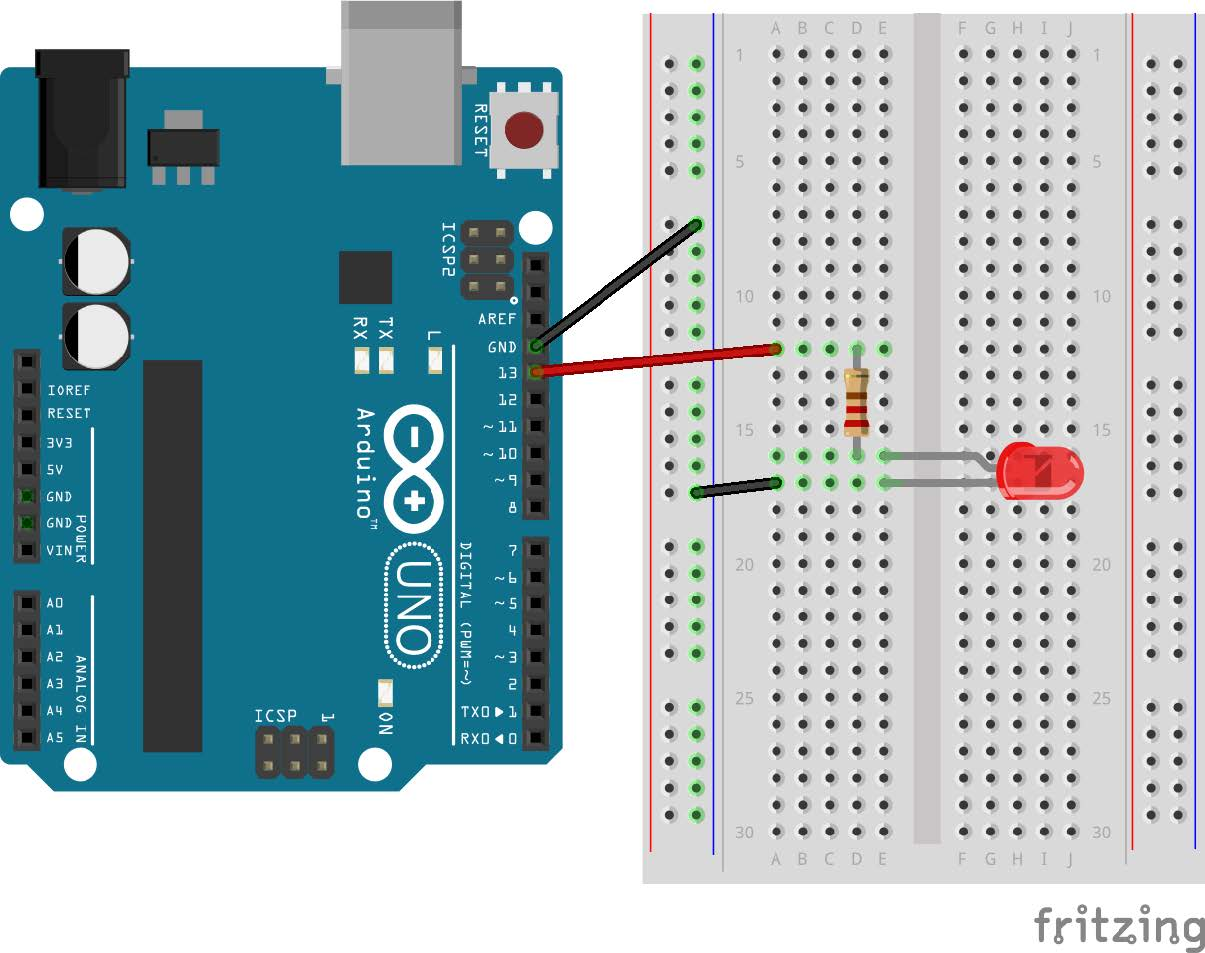tel. 24910 23755 / fax 24910 26620`/*`
`Turns on a LED on for one second, then off for`
`one second, repeatedly.`
`*/`
`// constants won't change. They're used here to`
`// set pin numbers:`
`// in Pin 13 we will connect a LED.`
`// Give it a name.`
`const int led = 13;`
`// the setup routine runs once when you press`
`// reset:`
`void setup() {`
`  // initialize the digital pin as an output.`
`  pinMode(led, OUTPUT);`
`}`
`// the loop routine runs over and over again`
`//forever:`
`void loop() {`
`  // turn the LED on (HIGH is the voltage level)`
`  digitalWrite(led, HIGH);`
`  // wait for 3 seconds`
`  delay(3000);`
`  // turn the LED off by making the voltage LOW`
`  digitalWrite(led, LOW);`
`  // wait for a second`
`  delay(1000);`
`}`

Κατηγορίες: Arduino